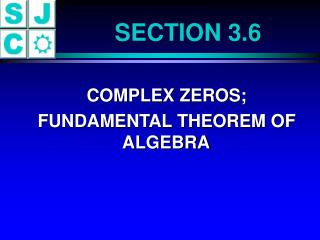DownloadDownload PresentationSECTION 3.6

# SECTION 3.6

Download Presentation## SECTION 3.6

- - - - - - - - - - - - - - - - - - - - - - - - - - - E N D - - - - - - - - - - - - - - - - - - - - - - - - - - -
##### Presentation Transcript

1. SECTION 3.6 • COMPLEX ZEROS; • FUNDAMENTAL THEOREM OF ALGEBRA

2. COMPLEX POLYNOMIAL FUNCTION A complex polynomial function f of degree n is a complex function of the form f(x) = a n x n + a n-1 x n-1 + . . . + a1x + a0 where an, a n-1, . . ., a1, a0 are complex numbers, an 0, n is a nonnegative integer, and x is a complex variable.

3. COMPLEX ZERO A complex number r is called a complex zero of a complex function f if f(r) = 0.

4. COMPLEX ZEROS We have learned that some quadratic equations have no real solutions but that in the complex number system every quadratic equation has a solution, either real or complex.

5. FUNDAMENTAL THEOREM OF ALGEBRA Every complex polynomial function f(x) of degree n  1 has at least one complex zero.

6. THEOREM Every complex polynomial function f(x) of degree n  1 can be factored into n linear factors (not necessarily distinct) of the form f(x) = an(x - r1)(x - r2)   (x - rn) where an, r1, r2, . . ., rn are complex numbers.

7. CONJUGATE PAIRS THEOREM Let f(x) be a complex polynomial whose coefficients are real numbers. If r = a + bi is a zero of f, then the complex conjugate r = a - bi is also a zero of f.

8. CONJUGATE PAIRS THEOREM In other words, for complex polynomials whose coefficients are real numbers, the zeros occur in conjugate pairs.

9. CORORLLARY A complex polynomial f of odd degree with real coefficients has at least one real zero.

10. EXAMPLE A polynomial f of degree 5 whose coefficients are real numbers has the zeros 1, 5i, and 1 + i. Find the remaining two zeros. - 5i 1 - i

11. EXAMPLE Find a polynomial f of degree 4 whose coefficients are real numbers and has the zeros 1, 1, and - 4 + i. f(x) = a(x - 1)(x - 1)[x - (- 4 + i)][x - (- 4 - i)] First, let a = 1; Graph the resulting polynomial. Then look at other a’s.

12. EXAMPLE It is known that 2 + i is a zero of f(x) = x4 - 8x3 + 64x - 105 Find the remaining zeros. - 3, 7, 2 + i and 2 - i

13. EXAMPLE Find the complex zeros of the polynomial function f(x) = 3x4 + 5x3 + 25x2 + 45x - 18

14. CONCLUSION OF SECTION 3.6